# Water at 10°C (ρ = 999.7 kg/m3 and μ = 1.307 × 10–3 kg/m·s) is flowing steadily in a 0.12-cm-diameter, 15-m-long pipe at an average velocity of 0.9 m/s. Determine (a) the pressure drop, (b) the head loss, and (c) the pumping power requirement to overcome this pressure drop.

Question-AnswerCategory: Fluid MechanicsWater at 10°C (ρ = 999.7 kg/m3 and μ = 1.307 × 10–3 kg/m·s) is flowing steadily in a 0.12-cm-diameter, 15-m-long pipe at an average velocity of 0.9 m/s. Determine (a) the pressure drop, (b) the head loss, and (c) the pumping power requirement to overcome this pressure drop.

Water at 10°C (ρ = 999.7 kg/m3 and μ = 1.307 × 10–3 kg/m·s) is flowing steadily in a 0.12-cm-diameter, 15-m-long pipe at an average velocity of 0.9 m/s. Determine (a) the pressure drop, (b) the head loss, and (c) the pumping power requirement to overcome this pressure drop.

Step: 1

Calculate the Reynolds number.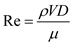Here,is the density of water, V is the average velocity of the water, D is the diameter of the pipe, andis the dynamic viscosity of the water.

Step: 2

Substitute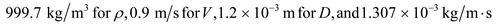.Here,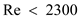therefore, the flow is laminar.

Step: 3

Calculate the friction factor.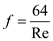Substitute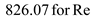.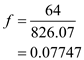Step: 4

a)
Calculate the pressure drop using the equation.Here, L is the length of the pipe.
Substitute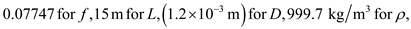and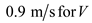.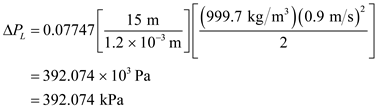Therefore, the pressure drop is.

Step: 5

b)
Calculate the head loss using the equation.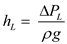Here, g is the gravitational acceleration.

Substitute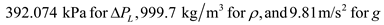.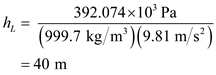Therefore, the head loss is.

Step: 6

c)
Calculate the pumping power to overcome the pressure drop.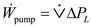…… (1)
Here,is the flow rate.
Calculate the flow rate.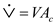…… (2)
Here,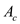is the cross sectional area of the flow.
Calculate the cross sectional flow area.SubstituteStep: 7

Substitute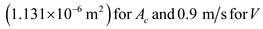in equation (2).Calculate the pumping power by substitute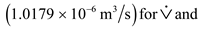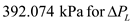in equation (1).Therefore, the pumping power required to overcome the pressure loss is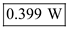.